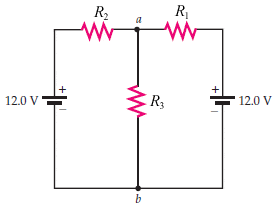# Two Batteries in a Circuit

henryg09Find the magnitude of the current in each resistor.
R1 = 2.40 Ω, R2 = 4.45 Ω, and R3 = 6.10 Ω.

I keep on attempting to use Kirchoff's Rules but it isn't working out. It doesn't click in my head if the current is flowing from the left battery, does the current still split? Is R3 double resistance in order to bring the current down to I?

Assuming there was another imaginary point right after the left terminal but before R2 and another point at a, I used the equation Va-Vb=(emf)-IR but since I don't know the Potential loss nor the current, I can't solve for either. Also placed more imaginary points mirrored to the right battery and set the Vb from both equations equal to each other but only thing I have left is 0=24V-4.45I1-6.85I2.. Grrr... Anyone give me a hint please?